# Finding Primes Like 1367

Ancient Greek Eratosthenes had a method of finding prime numbers. We call it the Sieve of Eratosthenes. You can use this method if you make a list of numbers, circle the first available prime number, cross out all of its multiples on the chart and repeat tat procedure over and over again. The next number that has not been crossed out is the next prime number. Numbers that get crossed out are composite numbers, and they can always be expressed as the product of prime numbers.

Many teachers have given their students a 100 chart to help them find the twenty-five prime numbers less than 100.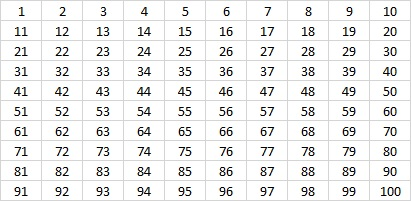If the number 1 were a prime number, we would have to cross out all of its multiples, and that would make 1 be the only prime number!?? That would be an unacceptable conclusion. It turns out that 1 cannot be either prime or composite. Perhaps you will want to put a star around it.

Here is a 100 chart with the multiples of 2, 3, 5, and 7 crossed out.Since 10 is a multiple of 2 and 5, it was easy to cross out their multiples. 9, a multiple of 3, is one less than 10, so 3’s multiples were also easy to cross out. Crossing out all the multiples of 7 is a little bit tedious at first, but you can even find a pattern for them as well.

Notice that the distance between multiples of any given prime is that prime number whether you count horizontally or vertically. The circled numbers AND all the numbers not crossed out on the chart are prime numbers.

This Sieve of Eratosthenes is a powerful method for finding prime numbers, but some of its power is lost when just a 100 chart is used. For pretty much the same amount of work, we could have used the list of numbers in a 10 × 12 chart because 10 × 12 = 120 which is one less than the next prime number squared, 11² = 121.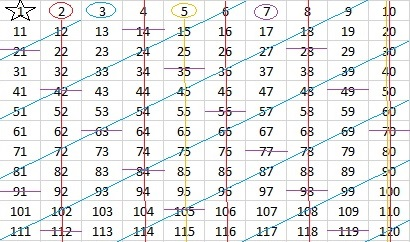But wait a minute! 11 is one more than 10 so its multiples would be SO easy to cross out. Let’s make the chart go to one less than 168 which is one less than 13²:Yeah, it’s not a perfect rectangle anymore, but for about the same effort, we get an additional fourteen prime numbers. I also like that all of these multiples of seven {21, 42, 63, 84, 105, 126, 147, 168} are sort of on a diagonal. What do you notice about the numbers in that set? The next number in the set isn’t on the chart, but can you figure out what it is and where it goes?

To find out if a number like 1367 is prime, I wouldn’t want to expand a 100 chart into a 1400 chart. That chart would also require me to cross out all the multiples of every prime number from 13 to 31, and that might be a big pain.  It’s much easier to see if 1367 is divisible by any of the prime numbers less than its square root. It isn’t, so I conclude:

• 1367 is a prime number.
• Prime factorization: 1367 is prime.
• 1367 has no exponents greater than 1 in its prime factorization, so √1367 cannot be simplified.
• The exponent in the prime factorization is 1. Adding one to that exponent we get (1 + 1) = 2. Therefore 1367 has exactly 2 factors.
• The factors of 1367 are outlined with their factor pair partners in the graphic below.How do we know that 1367 is a prime number? If 1367 were not a prime number, then it would be divisible by at least one prime number less than or equal to √1367. Since 1367 cannot be divided evenly by 2, 3, 5, 7, 11, 13, 17, 19, 23, 29 or 31, we know that 1367 is a prime number.

# 827 and Level 5

827 is one of the prime numbers in the fourth prime decade, (821, 823, 827, 829).

827 = 103 + 107+ 109 + 113+ 127 + 131 + 137, that’s the sum of 7 consecutive prime numbers.

Here’s today’s puzzle: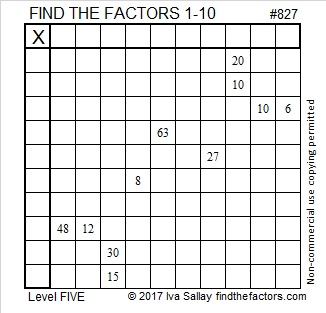Print the puzzles or type the solution on this excel file: 10-factors-822-828

• 827 is a prime number.
• Prime factorization: 827 is prime.
• The exponent of prime number 827 is 1. Adding 1 to that exponent we get (1 + 1) = 2. Therefore 827 has exactly 2 factors.
• Factors of 827: 1, 827
• Factor pairs: 827 = 1 × 827
• 827 has no square factors that allow its square root to be simplified. √827 ≈ 28.7576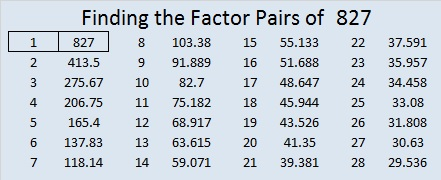How do we know that 827 is a prime number? If 827 were not a prime number, then it would be divisible by at least one prime number less than or equal to √827 ≈ 28.8. Since 827 cannot be divided evenly by 2, 3, 5, 7, 11, 13, 17, 19, or 23, we know that 827 is a prime number.# 809 Palindromes, Factors, Whoop-de-dooPrint the puzzles or type the solution on this excel file: 10-factors 807-814

Normally I would tell you that 809 is a palindrome in two different bases:

• 676 in BASE 11 because 6(121) + 7(11) + 6(1) = 809
• 575 in BASE 12 because 5(144) + 7(12) +5(1) = 809

But whoop-de-doo, all that really means is that (x – 11) is a factor of 6x² + 7x – 803, and (x – 12) is a factor of 5x² + 7x – 804.

Isn’t it just as exciting that ⁰¹²³⁴⁵⁶⁷⁸⁹

• (x – 2) is a factor of x⁹+ x⁸ + x⁵ + x³ – 808 because 809 is 1100101001 in BASE 2?
• (x – 3) is a factor of x⁶ + 2x³ + 2x² +2x – 807 because 809 is 1002222 in BASE 3?
• (x – 4) is a factor of 3x⁴ + 2x² + 2x – 808 because 809 is 30221 in BASE 4?
• (x – 5) is a factor of x⁴ + x³ + 2x² + x – 805 because 809 is 11214 in BASE 5?

Notice that the last number in each of those polynomials is divisible by the BASE number.

Palindromes NEVER end in zero so the polynomials they produce will NEVER end in the original base 10 number.

So are palindromes really so special? Today I am much more excited that figuring out what a number is in another base can give us a factor of a corresponding polynomial!

How do I know what those polynomials are? Let me use 809 in BASE 6 as an example:

Since 809 is 3425 in BASE 6, I know that

• 3(6³) + 4(6²) + 2(6¹) + 5(6º) = 809
• 3(216) + 4(36) + 2(6) + 5(1) – 809 = 0
• so 3(216) + 4(36) + 2(6) – 804 = 0
• thus (x – 6) is a factor of 3x³ + 4x² + 2x – 804 because of the factor theorem.

If I told you what 809 is in Bases 7, 8, 9, and 10 would you be able to write the corresponding polynomials that are divisible by (x – 7), (x – 8), (x – 9), and (x – 10) respectively?

• 2234 in BASE 7
• 1451 in BASE 8
• 1088 in BASE 9
• 809 in BASE 10

Scroll down past 809’s factoring information to see if you found the correct polynomials.

—————–

• 809 is a prime number.
• Prime factorization: 809 is prime.
• The exponent of prime number 809 is 1. Adding 1 to that exponent we get (1 + 1) = 2. Therefore 809 has exactly 2 factors.
• Factors of 809: 1, 809
• Factor pairs: 809 = 1 x 809
• 809 has no square factors that allow its square root to be simplified. √809 ≈ 28.4429253066558.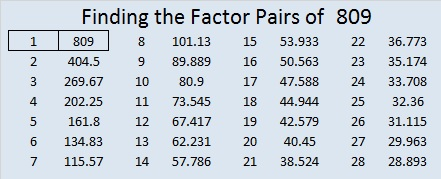How do we know that 809 is a prime number? If 809 were not a prime number, then it would be divisible by at least one prime number less than or equal to √809 ≈ 28.4. Since 809 cannot be divided evenly by 2, 3, 5, 7, 11, 13, 17, 19, or 23, we know that 809 is a prime number.

Here’s another way we know that 809 is a prime number: Since its last two digits divided by 4 leave a remainder of 1, and 28² + 5² = 809 with 28 and 5 having no common prime factors, 809 will be prime unless it is divisible by a prime number Pythagorean triple hypotenuse less than or equal to √809 ≈ 28.4. Since 809 is not divisible by 5, 13, or 17, we know that 809 is a prime number.

——————–

Were you able to find those polynomials from knowing what 809 is in other bases? Check your work with the answers below:

• 2234 Base 7 tells us (x – 7) is a factor of 2x³ + 2x² + 3x – 805
• 1451 Base 8 tells us (x – 8) is a factor of x³ + 4x² + 5x – 808
• 1088 Base 9 tells us (x – 9) is a factor of x³ + 8x – 801
• 809 Base 10 tells us (x – 10) is a factor of 8x² – 800

If you’ve made it this far, even if I’ve made you feel a little dizzy, you’ve done GREAT! Keep up the good work!# 792 Number Facts and Factors of the Year 2017

Happy New Year, everybody!

So much of what I’ve included in this post is a bit difficult for elementary school students, but here is an area problem that they should be able to do: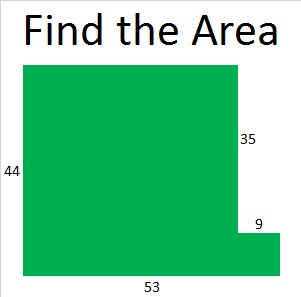Perhaps you’ll recognize that as just another way to illustrate that 44² + 9² = 2017.

This area problem based on 33² + 28² + 12² = 2017 will be a little bit more challenging for students: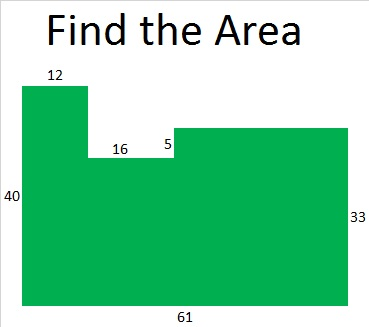2016 had more factors, positive and negative, than anybody could have imagined, but 2017 is a prime number year, so hopefully it will be filled with less drama.

I have a lot to say about the number 2017 with a little help from twitter.

2017 has only TWO positive real factors, 1 and 2017, and only TWO negative real factors, -1 and -2017. Positive or negative, ALL the real possible factor pairs for 2017 are

• 1 x 2017 and (-1) x (-2017).

2017 = 4(504) + 1. So 2017 is the sum of two squares. Which ones?

44² + 9² = 2017. That sum-of-squares number fact means that 2017 is the hypotenuse of a Pythagorean triple, specifically, 792-1855-2017. Since 2017 is a prime number, this triple is also a primitive.Here’s how those numbers were calculated from the fact that  44² + 9² = 2017:

• 2(44)(9) = 792
• 44² – 9² = 1855
• 44² + 9² = 2017

2017 is also the short leg in a rather monstrous primitive Pythagorean triple:

• 2017² + 2,034,144² = 2,034,145²

Hmm. 44² + 9² = 2017 means we can find some COMPLEX factor pairs for 2017:

• (44 + 9i)(44 – 9i) = 2017
• (-44 + 9i)(-44 – 9i) = 2017
• (9 + 44i)(9 – 44i) = 2017
• (-9 + 44i)(-9 – 44i) = 2017

Who knows what all those COMPLEX factors will bring to the coming year? Each of them was derived from the fact that 44² + 9² = 2017.

2017 is the sum of THREE squares three different ways:

• 37² + 18² + 18²  = 2017
• 33² + 28² + 12² = 2017
• 30² + 26² + 21² = 2017

Since 37² = 35² + 12², 30² = 24² + 18², and 26² = 24² + 10², we can write 2017 as the sum of these squares, too:

• 35² + 18² + 18² + 12² = 2017
• 30² + 24² + 21² + 10² = 2017
• 26² + 24² + 21² + 18² = 2017
• 24² + 24² + 21² + 18² + 10² = 2017

Here’s more sums and/or differences of squares from twitter:

This representation of 2017 is “two” powerful:

2017 is a PALINDROME in bases 31, 32, and 36:

• 232 BASE 31; note that 2(31²) + 3(31) + 2(1) = 2017
• 1V1 BASE 32 (V is 31 base 10); note that 1(32²) + 31(32) + 1(1) = 2017
• 1K1 BASE 36 (K is 20 base 10); note that 1(36²) + 20(36) + 1(1) = 2017

Also, OEIS.org tells us this 2017 fact about the totient function: “2017 is a value of n for which φ(n) = φ(n-1) + φ(n-2).”

PROOF that 2017 is a prime number:

We can use these three facts to help us verify that 2017 is a prime number:

1. 2017 = 4(504) + 1
2. 44² + 9² = 2017
3. √2017 ≈ 44.8998886

Since 2017 can be written as one more than a multiple of four, and 44 and 9 have no common prime factors, 2017 will be a prime number unless it is divisible by 5, 13, 17, 29, 37, or 41 (all the prime numbers less than √2017 ≈ 44.9 that have a remainder of one when divided by 4). That’s right, we ONLY have to divide 2017 by those SIX numbers to verify that it is prime: (Read here for why this is true.)

• 2017 ÷ 5 = 403.4
• 2017 ÷ 13 ≈ 155.15
• 2017 ÷ 17 ≈ 118.65
• 2017 ÷ 29 ≈ 69.55
• 2017 ÷ 37 ≈ 54.51
• 2017 ÷ 41 ≈ 49.20

We don’t get a whole number answer for any of those divisions, so 2017 is prime!

If you’re looking for more reasons to be interested in the number 2017, read David Radcliffe’s article:

Here are some other lovely mathematical thoughts about 2017 that I found on twitter:

In case you don’t know (and apparently a lot of people don’t know), 0! = 1, so this expression really does equal 2017.

And finally, if you click on this next link, David Mitchell will explain the tessellation of the number 2017.

——————————–

Since this is my 792nd post, I’ll write a little bit about the number 792:

792 has 24 factors so I’ll include a few of its many possible factor trees: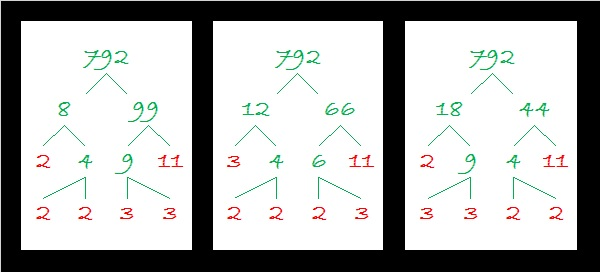Since 792 has so many factors, it is a leg in MANY Pythagorean triples. Here I list some factors that generate PRIMITIVE triples:

• 2(396)(1): 792-156815-156817
• 2(99)(4): 792-9785-9817
• 2(44)(9): 792-1855-2017 (illustrated near the top of this post)
• 2(36)(11): 792-1175-1417

792 is the sum of three squares four different ways including three ways that repeat squares:

• 28² + 2² + 2² = 792
• 26² + 10² + 4² = 792
• 20² + 14² + 14² = 792
• 18² + 18² + 12² = 792

792 is a palindrome in bases 32 and 35:

• OO BASE 32 (O is 24 base 10); note that 24(32) + 24(1) = 792
• MM BASE 35 (M is 22 base 10); note that 22(35) + 22(1) = 792

Finally here is the factoring information for the number 792:

• 792 is a composite number.
• Prime factorization: 792 = 2 x 2 x 2 x 3 x 3 x 11, which can be written 792 = (2^3) x (3^2) x 11
• The exponents in the prime factorization are 3, 2 and 1. Adding one to each and multiplying we get (3 + 1)(2 + 1)(1 + 1) = 4 x 3 x 2 = 24. Therefore 792 has exactly 24 factors.
• Factors of 792: 1, 2, 3, 4, 6, 8, 9, 11, 12, 18, 22, 24, 33, 36, 44, 66, 72, 88, 99, 132, 198, 264, 396, 792
• Factor pairs: 792 = 1 x 792, 2 x 396, 3 x 264, 4 x 198, 6 x 132, 8 x 99, 9 x 88, 11 x 72, 12 x 66, 18 x 44, 22 x 36 or 24 x 33

Taking the factor pair with the largest square number factor, we get √792 = (√36)(√22) = 6√22 ≈ 28.14249.# 787 Always a Unique Solution

• 787 is a prime number.
• Prime factorization: 787 is prime.
• The exponent of prime number 787 is 1. Adding 1 to that exponent we get (1 + 1) = 2. Therefore 787 has exactly 2 factors.
• Factors of 787: 1, 787
• Factor pairs: 787 = 1 x 787
• 787 has no square factors that allow its square root to be simplified. √787 ≈ 28.05352.Now for today’s puzzle….The fact that these Find the Factor puzzles always have a unique solution is an important clue in solving this rather difficult puzzle. Good luck!Print the puzzles or type the solution on this excel file: 12-factors-782-787

—————————————–

Here’s more about the number 787:

787 is a palindrome in bases 4, 10, 11 and 16:

• 30103 BASE 4; note that 3(256) + 0(64) + 1(16) + 0(4) + 3(1) = 787
• 787 BASE 10; note that 7(100) + 8(10) + 7(1) = 787
• 656 BASE 11; note that 6(121) + 5(11) + 6(1) = 787
• 313 BASE 16; note that 3(256) + 1(16) + 3(1) = 787

—————————————–

What did I mean when I wrote that the puzzles always having a unique solution is an important clue? There is only one clue in the puzzle that is divisible by 11. One of the rows and one of the columns do not have a clue, so the other 11 will go with one of them. The cell where the empty row and empty column intersect cannot be 132 because if that worked, it would produce two possible solutions to the puzzle. This table explains a logical order to find the solution.# 739 and Level 1

### Today’s Puzzle: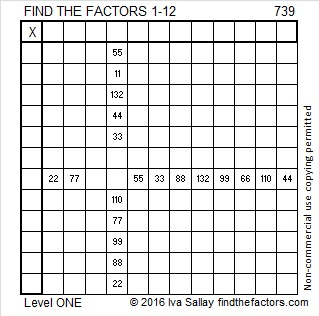Print the puzzles or type the solution on this excel file: 12 Factors 2016-01-11

### Factors of 739:

• 739 is a prime number.
• Prime factorization: 739 is prime.
• The exponent of prime number 739 is 1. Adding 1 to that exponent we get (1 + 1) = 2. Therefore 739 has exactly 2 factors.
• Factors of 739: 1, 739
• Factor pairs: 739 = 1 x 739
• 739 has no square factors that allow its square root to be simplified. √739 ≈ 27.1845544.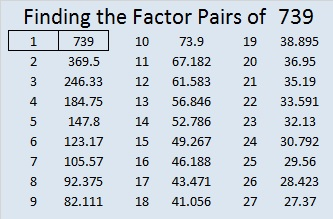How do we know that 739 is a prime number? If 739 were not a prime number, then it would be divisible by at least one prime number less than or equal to √739 ≈ 27.2. Since 739 cannot be divided evenly by 2, 3, 5, 7, 11, 13, 17, 19, or 23, we know that 739 is a prime number.

### More about the Number 739:

739 is prime so there is only one way it can be written as the sum of consecutive numbers:

• 369 + 370 = 739.

However, 739 can be written as the sum of 3 squares 3 different ways:

• 27² + 3² + 1² = 739
• 21² + 17² + 3² = 739
• 17² + 15² + 15² = 739

739 is included in this list of prime numbers.

### Factors for Today’s Puzzle:# 733 and Level 2

• 733 is a prime number.
• Prime factorization: 733 is prime.
• The exponent of prime number 733 is 1. Adding 1 to that exponent we get (1 + 1) = 2. Therefore 733 has exactly 2 factors.
• Factors of 733: 1, 733
• Factor pairs: 733 = 1 x 733
• 733 has no square factors that allow its square root to be simplified. √733 ≈ 27.0739727.

How do we know that 733 is a prime number? If 733 were not a prime number, then it would be divisible by at least one prime number less than or equal to √733 ≈ 27.1. Since 733 cannot be divided evenly by 2, 3, 5, 7, 11, 13, 17, 19, or 23, we know that 733 is a prime number.

Here’s another way we know that 733 is a prime number: Since  its last two digits divided by 4 leave a remainder of 1, and 17² + 2² = 733 with 17 and 2 having no common prime factors, 733 will be prime unless it is divisible by a prime number Pythagorean hypotenuse less than or equal to √733 ≈ 27.1. Since 733 is not divisible by 5, 13, or 17, we know that 733 is a prime number.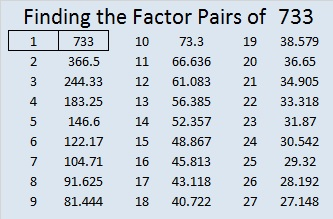Give this Level 2 factoring puzzle a try: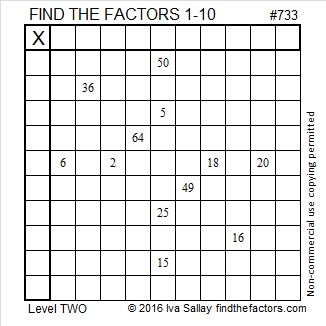Print the puzzles or type the solution on this excel file: 10 Factors 2016-01-04

———————————————————

Here are some more facts about the number 733:

733 is prime, so 366 + 367 = 733 is the only way it can be written as the sum of consecutive numbers.

733 is the sum of the prime numbers from 17 to 79. I think there’s a good chance you know what all those prime numbers are.

733 is also the sum of the five prime numbers from 137  to 157. You might not know what the missing primes are, but here’s a hint: If you subtract 100 from any of those three primes, you will get a composite number, and there are only three odd composite numbers that don’t end in 5 between 37 and 57.

27² + 2² = 733 so 733 is the hypotenuse of the (primitive) Pythagorean triple 108-725-733 which is calculated from 2(27)(2), 27² – 2², 27² + 2².

Thus, 108² + 725² = 733².

733 is palindrome 292 in BASE 17; note that 2(17²) + 9(17) + 2(1) = 733.

From Wikipedia I learned two other interesting facts about the number 733:

• 727, 733, 739 are consecutive prime numbers whose average is 733.  That makes 733 the 13th balanced prime. It is exactly the same distance from the previous prime and the prime that follows it.
• 337, 373, 733 are three of only nine 3-digit permutable prime numbers. No matter their order, those exact 3 digits produce a prime number.

———————————————————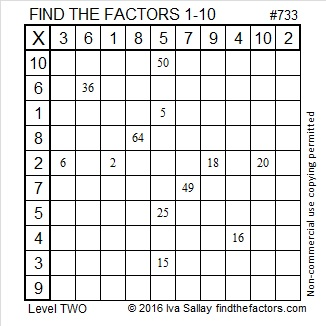# 727 Enjoy Some Sparkling Cider!

Have a happy New Year’s Eve! My husband and I will be enjoying some Sparkling Apple-Pear. I invite you to have some, too.Perhaps the goblet in the puzzle can start off your festivities.Print the puzzles or type the solution on this excel file: 12 Factors 2015-12-28

—————————————————————————————————

• 727 is a prime number.
• Prime factorization: 727 is prime.
• The exponent of prime number 727 is 1. Adding 1 to that exponent we get (1 + 1) = 2. Therefore 727 has exactly 2 factors.
• Factors of 727: 1, 727
• Factor pairs: 727 = 1 x 727
• 727 has no square factors that allow its square root to be simplified. √727 ≈ 26.9629375.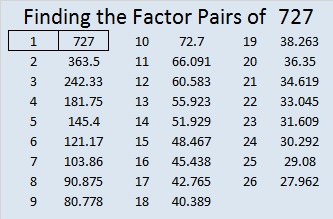727 is a palindrome in base 10 and one other base:

• 727 BASE 10; note that 7(100) + 2(10) + 7(1) = 727.
• 1B1 BASE 22 (B = 11 base 10); note that 1(22²) + 11(22) + 1(1) = 727.

OEIS.org informs us that 727² = 528529, a number whose digits can be split in half to make two consecutive numbers.

Since 727 is a prime number, there is only one way it can be expressed as the sum of consecutive numbers: 363 + 364 = 727.

—————————————————————————————————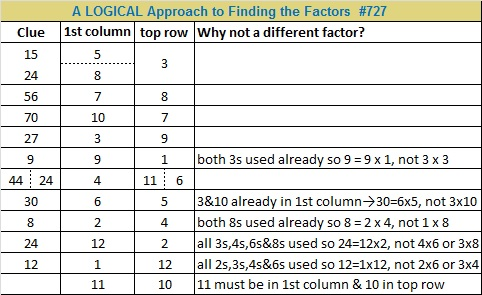# 719 and Level 3

• 719 is a prime number.
• Prime factorization: 719 is prime.
• The exponent of prime number 719 is 1. Adding 1 to that exponent we get (1 + 1) = 2. Therefore 719 has exactly 2 factors.
• Factors of 719: 1, 719
• Factor pairs: 719 = 1 x 719
• 719 has no square factors that allow its square root to be simplified. √719 ≈ 26.814175.

How do we know that 719 is a prime number? If 719 were not a prime number, then it would be divisible by at least one prime number less than or equal to √719 ≈ 26.8. Since 719 cannot be divided evenly by 2, 3, 5, 7, 11, 13, 17, 19, or 23, we know that 719 is a prime number.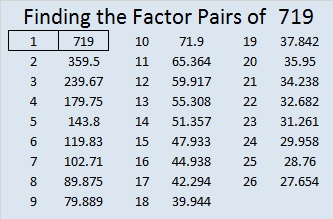Here is today’s puzzle: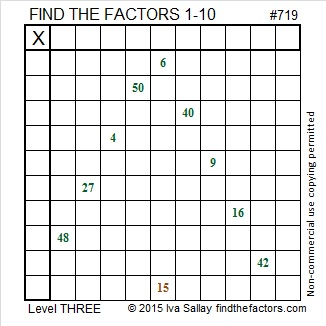Print the puzzles or type the solution on this excel file: 10 Factors 2015-12-21

—————————————————————————————

Here’s a little more about the number 719:

719 is the sum of the prime numbers from 89 to 113. Can you name those seven prime numbers?

719 is also a palindrome in two other bases:

• 878 BASE 9; note that 8(81) + 7(9) + 8(1) = 719
• 434 BASE 13; note that 4(169) + 3(13) + 4(1) = 719

—————————————————————————————

A Logical Approach to solve a FIND THE FACTORS puzzle: Find the column or row with two clues and find their common factor. (None of the factors are greater than 10.)  Write the corresponding factors in the factor column (1st column) and factor row (top row).  Because this is a level three puzzle, you have now written a factor at the top of the factor column. Continue to work from the top of the factor column to the bottom, finding factors and filling in the factor column and the factor row one cell at a time as you go.# 661 Candy Corn

25² + 6² = 661

661 is the hypotenuse of the primitive Pythagorean triple 300-589-661 which was calculated using 2(25)(6), 25² – 6², 25² + 6².

661 is also the sum of the three prime numbers from 211 to 227. What is the prime number in the middle of the sum?

This Find the Factors puzzle is supposed to look like a piece of candy corn. 🙂Print the puzzles or type the solution on this excel file: 10 Factors 2015-10-26

Here’s the puzzle without the possibly distracting color: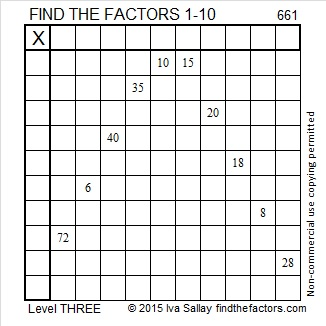————————————

• 661 is a prime number. 659 and 661 are twin primes.
• Prime factorization: 661 is prime.
• The exponent of prime number 661 is 1. Adding 1 to that exponent we get (1 + 1) = 2. Therefore 661 has exactly 2 factors.
• Factors of 661: 1, 661
• Factor pairs: 661 = 1 x 661
• 661 has no square factors that allow its square root to be simplified. √661 ≈ 25.70992.How do we know that 661 is a prime number? If 661 were not a prime number, then it would be divisible by at least one prime number less than or equal to √661 ≈ 25.7. Since 661 cannot be divided evenly by 2, 3, 5, 7, 11, 13, 17, 19, or 23, we know that 661 is a prime number.

Here’s another way we know that 661 is a prime number: Since 25² + 6² = 661, and 25 and 6 have no common prime factors, 661 will be prime unless it is divisible by a primitive Pythagorean hypotenuse less than or equal to √661 ≈ 25.7. Since 661 is not divisible by 5, 13, or 17, we know that 661 is a prime number.

—————————————————————————————————

A Logical Approach to solve a FIND THE FACTORS puzzle: Find the column or row with two clues and find their common factor. (None of the factors are greater than 10.)  Write the corresponding factors in the factor column (1st column) and factor row (top row).  Because this is a level three puzzle, you have now written a factor at the top of the factor column. Continue to work from the top of the factor column to the bottom, finding factors and filling in the factor column and the factor row one cell at a time as you go.